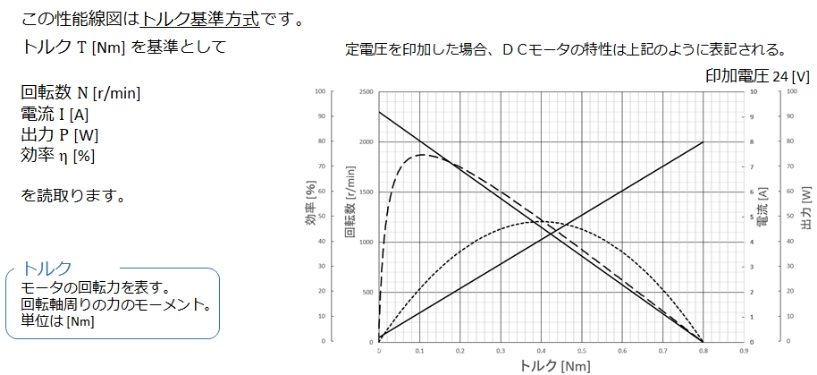TOP > English > Product Information > How to Read Performance Curves

## How to Read Performance Curves

### Unit Conversion

 Torque 1gf-cm=0.098mNm=0.014oz-in 1mNm=10.2gf-cm=0.142oz-in 1oz-in=7.056mNm=72.0gf-cm Length 1mm=0.039in 1in=25.4mm Weight 1g=0.035oz 1oz=28.35g

### How to Read Motor Performance

#### ■Revolutions: N

The number of revolutions (unit: rpm) is indicated with a straight line. The revolution line shifts laterally depending on voltage increases or decreases.

#### Current: I

The current (unit: A) is indicated by a straight line, from no load condition to motor lock.

Current at lock (Is)

#### ■Torque: T

The torque (unit: gf-cm) is the load borne by the motor shaft.
Choose an appropriate load for the motor.

Rated torque (Tn)  Lock torque (Ts)

#### ■Efficiency：η

The efficiency (unit: %) is calculated from the input and output values. To maximally leverage the performance of a motor, it should be used at near-peak efficiency.

Efficiency （η）＝Output / input×100
Input（W）＝motor terminal voltage （E）×current（I）

#### ■Output：P

The output (unit: W) is the amount of mechanical energy output by the motor. It is calculated using the following formula:

Output（P）＝1.027×N×T×10-5